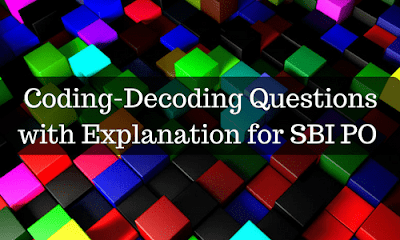New Students Offer - Use Code HELLO

# Coding-Decoding Questions for SBI PO Exam(Q1-Q5) Study the following information carefully and answer the questions given below:

#### In a certain code language

• ‘Bank Exams Today Website’ is written as ‘@5X \$7E #5O !4A’
• ‘Provides Free Materials To’ is written as ‘#2O %8R &9A ^4R’
• ‘Prepare For Bank Exams ’ is written as ‘@5X %7R !4A ^3O ’
• ‘Use Today Enjoy Tomorrow’ is written as ‘ #5O *3S #8O @5N’

1. Which of the following is code for ‘Exams’?
A. #5O
B. @5N
C. \$7E
D. @5X
E. None of these

2. Which of the following is code for ‘Use’?
A. *3E
B. ^3O
C. *3S
D. #5O
E. None of these

3. In the given code language, what does the code ‘#8O’ means?
A. Tomorrow
B. Today
C. Provides
D. Enjoy
E. None of these

4. Which of the following is the code for ‘Use For Free Today’?
A. #5O *3S *4R ^3O
B. *3S ^3O ^4R @5N
C. *3S *3O ^4R @5N
D. #5O *3S ^4R ^3O
E. None of these

5. In the given code language, what does the code ‘Bank Materials For SBI ’ means?
A.!4A &9A ^3O @3B
B. &9A *3S +3B ^4R
C. ^3O +3B &9A !4A
D. ^4R +3B &9A ^3O
E. None of these

1. D
2. C
3. A
4. D
5. C

#### Concept Explanation:

• Each code has symbol, Number and a Letter
• Now when we analyze the code, each of them has a number in the middle, which implies the total number of letters of that corresponding word.
• Letter in the end represents the second letter of the corresponding word
• Finally we have to find the logic behind the symbols used
• By taking last line into consideration, symbol ‘#’ comes twice, which clearly implies it encrypt the letter ‘T’
• By comparing 1st line with 3rd line, words ‘Bank Exams’ get repeated, from which we can conclude
• ! Represents B
•  @ Represents E
• Similarly using above logic we can easily find the corresponding codes for all the word.

(Q6-Q10) Study the following information carefully and answer the questions given below:

#### In a certain code language

• ‘Apple Cat Dog Beetroot’ is written as ‘*T8 ^T3 @E5 %G3’
• ‘Baroda Axis Canara Dena ’ is written as ‘%A4 @S4 ^A6 *A6’
• ‘Decoding Aptitude Coding Basel ’ is written as ‘@E8 %G8 *L5 ^G6’
• ‘Cousin Aunty Brother Dear’ is written as ‘^N6 %R4 *R7 @Y5’

6. Which of the following code for ‘Axis’?
A. ^A6
B. *A6
C. @S4
D. %A4
E. None of these

7. Which of the following code for ‘Cousin’?
A. ^G6
B. ^N6
C. *R7
D. %G3
E. None of these

8. In the given code language, what does the code ‘^A6’ means?
A. Baroda
B. Cousin
C. Beetroot
D. Canara
E. None of these

9. Which of the following is the code for ‘Apple’?
A. @E5
B. *A6
C. *L5
D. @Y5
E. None of these

10. What may be the possible code for ‘SBI’ according to the above coding concept?
A. %I3
B.!I3
C.@I3
D.*I3
E. ^T3

6. C
7. B
8. D
9. A
10. B

#### Concept Explanation:

• Each code has symbol, Letter and a number
• Same symbols(@,*,%,^) get repeated for every line, similarly Words starts with same set of alphabets (A,B,C,D) for every line, which clearly says each letter corresponds to a particular symbol.
• Now when we analyze the code, each of them ends with a number, which implies the total number of letters of that corresponding word.
• Finally letter in the middle represents the end letter of the corresponding word
• By the above concept decrypted code for first line will be
• Apple: @E5
• Cat: ^T3
• Dog: %G3
• Banana: *A6
• Therefore, Corresponding symbol for the alphabets will be
• A: @
• B: *
• C: ^
• D: %
• When we look at 5th Question, ‘SBI’ will also start with a symbol, but it will be other than the above-mentioned four and 2nd, 3rd positions of the code will be ‘I3’, therefore from the given option ‘!I3’ will be the possible code for ‘SBI’.

### Points to remember:

• If difficult coding-decoding question appears, try to find out the logic behind.
• If you can able to find the logic, it will take just a few seconds to find out the answers.